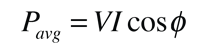# Power Factor

For a DC circuit the power is P=VI, and this relationship also holds for the instantaneous power in an AC circuit. However, the average power in an AC circuit expressed in terms of the rms voltage and current iswhere φ is the phase angle between the voltage and current. The additional term is called the power factorFrom the phasor diagram for AC impedance, it can be seen that the power factor is R/Z. For a purely resistive AC circuit, R=Z and the power factor = 1.

 Why is the power factor important?
Index

AC Circuits

 HyperPhysics***** Electricity and Magnetism R Nave
Go Back

# Importance of Power Factor

A power factor of one or "unity power factor" is the goal of any electric utility company since if the power factor is less than one, they have to supply more current to the user for a given amount of power use. In so doing, they incur more line losses. They also must have larger capacity equipment in place than would be otherwise necessary. As a result, an industrial facility will be charged a penalty if its power factor is much different from 1.

Industrial facilities tend to have a "lagging power factor", where the current lags the voltage (like an inductor). This is primarily the result of having a lot of electric induction motors - the windings of motors act as inductors as seen by the power supply. Capacitors have the opposite effect and can compensate for the inductive motor windings. Some industrial sites will have large banks of capacitors strictly for the purpose of correcting the power factor back toward one to save on utility company charges.

Index

AC Circuits

 HyperPhysics***** Electricity and Magnetism R Nave
Go Back